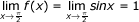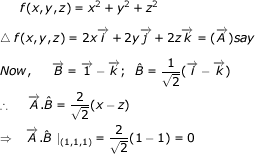# Calculus - Calculus MCQ

26:

The minimum value of | x2-5x+21 | is

 A. -5 B. 0 C. -1 D. -2 Answer Report Discuss Option: B Explanation : Since the value of a mod function cannot be less than zero, therefore    f(x) = | x2-5x+2 | is zero. Click on Discuss to view users comments. Write your comments here:
27:

Sum of the perimeters of a circle and a square is 1. If sum of the area is least, then

 A. side of the square is double the radius of circle B. side of the square is 1/2 of the radius of the circle C. side of the square is equal to radius of the circle D. none of these Answer Report Discuss Option: A Explanation :Click on Discuss to view users comments. Write your comments here:
28:

The maximum slope of the curve
-x3 + 6x3 + 2x + 1 is

 A. 14 B. 16 C. 19 D. -13 Answer Report Discuss Option: A Explanation : Let f(x) = -x3 + 6x2 + 2x + 1 Slope of the function is,       f'(x)  =  -3x2 + 12x + 2 = F(x) (say) Now to find minimum or maximum of F(x) Therefore                  F'(x) = - 6x + 12                                   F"(2) = -6 For maxima and minima, F'(x) = 0 ⇒                                x = 2  Therefore                   F"(2) = -6  Hence F(x) (slope) is maximum at x = 2   Maximum slope = F(2) = -3(2)2 + 12(2) + 2 = 14   Click on Discuss to view users comments. Write your comments here:
29:

Limit of the following series as x approaches is

 A. 2Π / 3 B. Π / 2 C. Π / 3 D. 1 Answer Report Discuss Option: D Explanation :Click on Discuss to view users comments. Write your comments here:
30:

Directional derivative of f ( x,  y,  z ) = x2 + y2 + z2 at the point (1, 1, 1) in the direction i - k is

 A. 0 B. 1 C. √2 D. 2√2 Answer Report Discuss Option: A Explanation :Click on Discuss to view users comments. Write your comments here: